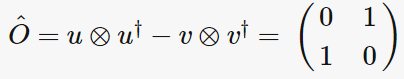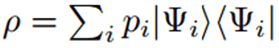# Finding the density matrix

• andphy
In summary, to find the density operator in a given Hilbert space, you first need to reconstruct the operator using a given orthonormal basis. Once you have the operator, you can use the completeness relation to find the eigenvalues and eigenvectors. The eigenvalues are non-negative and sum to 1, while the eigenvectors are linearly independent.

#### andphy

Hi,

How do eigenvalues and eigenvectors relate to the density operator. Given the eigenvalues of a matrix, can they help to find the density operator ?

I have seen the formula within http://www.quantiki.org/wiki/Density_matrix but given a matrix how do I work it out to get the density operator ?

Links to related useful information/example appreciated.

Thanks

Last edited:
From any operator you get a matrix when using a basis. If you have a given matrix you need to tell the basis to reconstruct the operator. In QT you have a Hilbert space, and usually you work with a orthonormal basis, ##|u_k \rangle##, ##k \in \mathbb{N}## or also quite often in a "generalized basis" when you describe the Hilbert space in terms of wave functions like in position or momentum representation, but let's stick to the simpler case of a discrete orthonormal basis; for a single particle in non-relativistic QT you can take the harmonic-oscillator energy eigenstates as a nice example.

Now given an operator ##\hat{O}##, you can write it with help of the basis in terms of its "matrix elements",
$$O_{jk}=\langle u_j|\hat{O} u_k \rangle.$$
The orthonormal set is also complete, i.e., you can write the unit operator using the completeness relation:
$$\sum_{k=1}^{\infty} |u_k \rangle \langle u_k \rangle=\hat{1}.$$
Using this completeness relation twice, you can easily reconstruct the operator using the same basis taken to evaluate its matrix elements:
$$\hat{O}=\sum_{j,k=1}^{\infty} |u_j \rangle \langle u_j|\hat{O} u_k \rangle \langle u_k|=\sum_{j,k=1}^{\infty} |u_j \rangle \langle{u}_k| O_{jk}.$$
The most convenient set for such a basis is to choose an eigenbasis of the operator in question. If the operator is self-adjoint (as is the case for the density operator), the corresponding eigenvectors can be made to an orthornomal set. Then you have
$$\hat{O} |u_j \rangle=o_j |u_j \rangle,$$
where ##o_j## is a eigen value of the operator and ##u_j## a correspondin eigenvector. Then the matrix elements simplify very much:
$$o_{jk} = \langle u_j|\hat{O} u_k \rangle = o_k \langle u_j|u_k \rangle=o_k \delta_{jk}.$$
Note that here no Einstein summation convention is applied! This tells you that in the eigenbasis the matrix representing the operator is diagonal with the eigenvalues as entries on the diagonal.

Then one of the sum in the general decomposition formula can be done, and it simplifies to
$$\hat{O}=\sum_{jk=1}^{\infty} |u_j \rangle \langle u_k | o_{jk} = \sum_{jk=1}^{\infty} |u_j \rangle \langle u_k | o_k \delta_{jk}=\sum_{j=1}^{\infty} |u_j \rangle \langle u_j| o_j.$$
All this applies to the density operator (a better name is "statistical operator"). Then the eigen values are by definition non negative and their sum adds to 1, but that doesn't change the above given general framework.

Thank You - so if I have eigenvalues of 1 and -1 and eigenvectors of 1/√2(1,1) and /√2(1,-1) - how do I calculate the density matrix ?

How can a density matrix (statistical operator) have negative eigenvalues?

Anyway with the given information you can reconstruct the operator wrt. the basis the eigenvectors are given as follows. First write the eigenvectors as column vectors
##u=\frac{1}{\sqrt{2}} \begin{pmatrix} 1 \\ 1 \end{pmatrix}, \quad v=\frac{1}{\sqrt{2}} \begin{pmatrix} 1 \\ -1 \end{pmatrix}.##
Then the operator is
##\hat{O}=u \otimes u^{\dagger} - v \otimes v^{\dagger}=\begin{pmatrix}0 & 1 \\ 1 & 0 \end{pmatrix}.##

Alternatively, you can also try to find a set of linearly independent density matrices and use their squareroot as basis vectors for the construction of a Hilbert space. In this way the expectation value of an operator A for a given mixed state can be calculated in the usual way: ##\langle \rho_1^{1/2}| A|\rho_1^{1/2}\rangle:=\mathrm{Tr} (\rho_1^{1/2} A \rho_1^{1/2})=\mathrm{Tr} ( A \rho_1)##But this means that Pauli-X matrice and respective density matrice result in the same matrice !

Perhaps I need to read more as still a bit confused on how density matrices are calculated given for instance the Pauli operators and their eigenvalues.

Again, note that ##\hat{O}## cannot be a density matrix, because this must be a positive semidefinite self-adjoint matrix (i.e., its eigen values must be ##\geq 0##) and its trace must be 1 (the trace is given by the sum of the matrix's diagonal elements or the sum of its eigenvalues, which is always the same).

ok... this s the formula for finding the density matrix:@vanhees71
Although this is an old post, but I really have to ask:

Why are you constructing the density matrix using the eigenvalues and not the probabilities? This makes it like if the eigenvalue has units of energy, the density matrix will have units of energy too... isn't the density matrix unitless and represents fractions of the system?

If you have a positive definite self-adjoint trace-class operator, you have statistical operator. The density matrix is this operator in the position representation
$$\rho(\vec{x},\vec{x}')=\langle \vec{x} |\hat{\rho}|\vec{x}' \rangle.$$
It has positive semidefinite eigenvalues and a corresponding complete orthonormal basis. The eigenvalues are the probabilities that the system is found in the corresponding eigenstates when measuring a corresponding observable.

The density operator is dimensionless, as can be seen from the normalization condition,
$$\mathrm{Tr} \hat{\rho}=1.$$
The density matrix has dimension ##1/\text{length}^3##, because for any observable
$$\langle O \rangle=\mathrm{Tr}(\hat{\rho} \hat{O})=\int_{\mathbb{R}^3} \mathrm{d}^3 \vec{x} \langle \vec{x}|\hat{\rho} \hat{O} \vec{x} \rangle = \int_{\mathbb{R}^3} \mathrm{d}^3 \vec{x} \int_{\mathbb{R}^3} \mathrm{d}^3 \vec{x}' \langle \vec{x}|\hat{\rho} \vec{x}' \rangle \langle \vec{x}'|\hat{O} \vec{x} \rangle = \int_{\mathbb{R}^3} \mathrm{d}^3 \vec{x} \int_{\mathbb{R}^3} \mathrm{d}^3 \vec{x}' \rho(\vec{x},\vec{x}') O(\vec{x}',\vec{x}).$$
Another way to see this is to realize that because of
$$\langle \vec{x}|\vec{x}' \rangle=\delta^{(3)}(\vec{x}-\vec{x}')$$
##|\vec{x} \rangle## is of dimension ##\mathrm{length}^{-3/2}##.

@vanhees71
I see. You're talking about the eigenvalues of the density matrix itself. I think the asker meant the eigenvalues of the Hamiltonian, which explains why he provided eigenvalues -1 and 1.

The problem is that the question is not well defined. Which "matrix"? If I reread the original posting #1 in your sense, it means you have an observable ##O##, described by a self-adjoint operator ##\hat{O}##, and now you take the trace using the eigenvectors of ##\hat{O}## then, you get, of course
$$\langle O \rangle = \sum_j \langle o_j |\hat{\rho} \hat{O} o_j \rangle=\sum_{j} o_j \langle o_j |\hat \rho o_j \rangle,$$
which shows that
$$P_j=\langle o_j |\hat{\rho} o_j \rangle$$
is the probability that the system is prepared in the pure state described by ##|o_j \rangle \langle o_j|##.

@vanhees71
I agree the asker asked an ambiguous question. The probability to be in state j is $P_j=\langle \psi | o_j \rangle$ I would say. I think people learning QM always get confused because the concept of preparation of states isn't clear. I guess a logical question for a new learner is "where the hell will I get $\psi$ from?"

That's a dangerous way to express it, because you have to distinguish between the (pure) states, described by normalized vectors in Hilbert space and the (generalized) eigenvectors of the self-adjoined operators representing observables. Their time evolution is different, depending on the chosen picture of time evolution. E.g., in the Schrödinger pictures the state vectors time-evolve according to the full Hamiltonian and the operators representing observables and thus also their eigenvectors are time-independent. In the Heisenberg picture it's the other way around, and often you use a general Dirac picture (e.g., the interaction picture in scattering theory). Only the correct expressions give the picture-independent observable quantities, i.e., probablity distributions of quantum theory, namely

If the system is prepared in a pure state represented by the normalized Hilbert-space vector ##|\psi \rangle##, then the proability (density) to find a value ##o_j## of the observable ##O## is given by
$$P_j=|\langle o_j|\psi \rangle|^2.$$
It is important to keep in mind that for the probability amplitude you always have the (generalized) scalar product between an eigenvector of an operator representing an observable and the state vector.

In the more general case of a mixed state, this mixed state is represented by a Statistical operator (a self-adjoint postive semi-definite trace-class operator ##\hat{\rho}## with ##\mathrm{Tr} \hat{\rho}=1##. Then the probability to find the value ##o_j## when measuring the observable ##O## is given by
$$P_j=\langle o_j|\hat{\rho} o_j \rangle.$$

The pure state can be subsumed in this general description. In this case the Statistical operator is given by the projection operator
$$\hat{\rho}=\hat{P}_{\psi}=|\psi \rangle \langle \psi|.$$